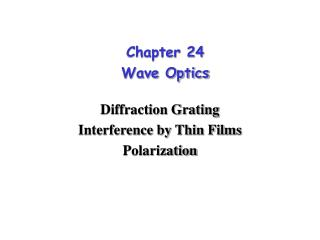DownloadDownload PresentationChapter 24 Wave Optics

Chapter 24 Wave Optics

Download PresentationChapter 24 Wave Optics

- - - - - - - - - - - - - - - - - - - - - - - - - - - E N D - - - - - - - - - - - - - - - - - - - - - - - - - - -
Presentation Transcript

1. Chapter 24Wave Optics Diffraction Grating Interference by Thin Films Polarization

2. Q d Q Extra distance ml sinQ=ml/d or dsinQ=ml m=0,1,2,3, . . . Constructive inference m=1/2,3/2,5/2, . . . Destructive inference

3. Example: In a double-slit experiment it is found that blue light of wavelength 460 nm gives a second-order maximum at a certain location on the screen. What wavelength of visible light would have a minimum at the same location? Answer:For constructive interference d sinQ=ml=2x460nm=920nm For destructive interference of the other light, we have d sinQ=(m’+1/2)l When the two angle are equal, then 920nm=(m’+1/2)l l=1.84x103 nm for m’=0 l=613 nm for m’=1 l=368 nm for m’=2 The only wavelength here that is visible is 613 nm

4. When a light wave travels from one medium to another, its frequency does not change, but its wavelength does: l2/l1=v2t/v1t=v2/v1=n1/n2 (v=c/n) n1/n2 = l2/l1= sin(r)/sin(i) The shorter l, the larger refraction angle

5. 1 mm 0.589 mm 1 m Example: Monochromatic yellow light illuminates two narrow slits 1 mm apart the screen is 1 m from the slits, and the distance from the central bright light to the next bright line is found to be 0.589 mm. Find the wave length of the light. Solution:d sinQ=ml, where m=1or l=d sinQ/1= (1x10-3m)(5.89x10-4m)/1m= 5.89x10-7 m =589 nm

6. There are two difficulties in using a double slit for measuring wavelengths. 1. The bright lines on the screen are actually extremely faint and an intense light source is therefore required; 2. The lines are relatively broad and it is hard to locate their center accurately.

7. Diffraction Grating A diffraction grating that consists of a large number of parallel slits overcomes both of these difficulties. A diffraction grating uses interference to disperse light. It is often an important component in optical instrumentation for wavelength determinations.

8. dsinQ=ml m=0,1,2,3, . . . Constructive inference m=1/2,3/2,5/2, . . . Destructive inference

9. For a diffraction grating, the intensity falls away from these maxima much more rapidly than that for a double slit. Because there are so many slits to act as sources, any angle other than those for maxima will be dark or nearly dark.

10. Example:Visible light includes wavelengths from 4x10-7 m to 7x10-7m. Find the angular width of the first-order spectrum produced by a grating ruled with 800 lines/cm. Solution: The slit space d that corresponding to 800 line/cm is d=(10-2 m/cm)/(8x103 lines/cm)=1.25x10-6 m Since m=1, sinQb=lb/d = 4x10-7m/1.25x10-6m = 0.32, Qb=19o sinQr=lr/d = 7x10-7m/1.25x10-6m = 0.56, Qr=34o The total width of the spectrum is therefore 34o-19o=15o The angle can be measured to very high accuracy, so the wavelength of a line can be determined to high accuracy using l=d sinQ /m

11. Question: A characteristic property of the spectra produced by a diffraction grating is • the sharpness of the bright lines • diffuseness of the bright lines • absence of bright lines • absence of dark lines Answer: a

12. Question: The greater the number of lines that are ruled on a grating of given width, • The shorter the wavelengths that can be diffracted • The longer the wavelengths that can be diffracted • The narrower the spectrum that is produced • The broader the spectrum that is produced Answer: d

13. Spectrometer and Spectroscopy using a grating or prism

14. A prism also disperses light n1/n2 = l2/l1= sin(r)/sin(i)

15. Question: White light strikes (a) a diffraction grating, and (b) a prism. A rainbow appears on a screen just below the direction of horizontal incident beam in each case. What is the color of the top of the rainbow in each case? Answer: (a) Violet for diffraction grating (ml=dsinQ) (b) Red for prism (n1/n2 = l2/l1)

16. Single-slit Diffraction Light from all parts of the slit travels the same distance and arrives "in phase" so there is a bright central maximum.

17. A Single-slit Diffraction Intensity A single slit diffraction pattern has a bright central maximum surrounded by much smaller maxima.